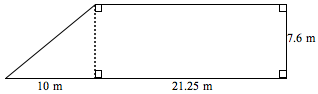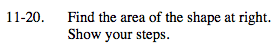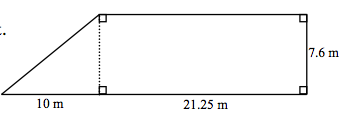Home > MC2 > Chapter 11 > Lesson 11.1.2 > Problem11-20

11-20.

Find the area of the shape below. Show your steps. Homework Help ✎There are two ways to find the area: by using the equation for finding the area of a trapezoid, or by noticing that the shape is made up of a triangle and a rectangle.

To find the perimeter start by finding the hypotenuse of the triangle by using the Pythagorean Theorem.

Divide the shape into two portions and use two different equations for finding the area for each shape:

$\text{Triangle: Area }= \frac{1}{2}(\text{base)(height})$

Rectangle: Area = (base) (height)

Add the two areas together to get your total area.

$\text{Triangle: area}=\frac{1}{2}(10\text{m})(7.6\text{m})$

$\text{Rectangle:area}=(21.25\text{m})(7.6\text{m})$